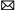# Three Phase AC Motors

The main difference between AC and DC motors is that the magnetic field generated by the stator rotates in the case of AC motors. A rotating magnetic field is key to the operation of all AC motors. The principle is simple. A magnetic field in the stator is made to rotate electrically around and around in a circle. Another magnetic field in the rotor is made to follow the rotation of this field pattern by being attracted and repelled by the stator field. Because the rotor is free to turn, it follows the rotating magnetic field in the stator.
It illustrates the concept of a rotating magnetic field as it applies to the stator of a three-phase AC motor. The operation can be summarized as follows:
• Three sets of windings are placed 120 electrical degrees apart with each set connected to one phase of the three-phase power supply.
• When three-phase current passes through the stator windings, a rotating magnetic field effect is produced that travels around the inside of the stator core.
• Polarity of the rotating magnetic field is shown at six selected positions marked off at 60 degree intervals on the sine waves representing the current flowing in the three phases, A, B, and C.
• In the example shown, the magnetic field will rotate around the stator in a clockwise direction.
• Simply interchanging any two of the three-phase power input leads to the stator windings reverses direction of rotation of the magnetic field.
• The number of poles is determined by how many times a phase winding appears. In this example, each winding appears twice, so this is a two-pole stator.

There are two ways to define AC motor speed. First is synchronous speed. The synchronous speed of an AC motor is the speed of the stator's magnetic field rotation. This is the motor's ideal theoretical, or mathematical, speed, since the rotor will always turn at a slightly slower rate. The other way motor speed is measured is called actual speed. This is the speed at which the shaft rotates. The nameplate of most AC motors lists the actual motor speed rather than the synchronous speed.

Rotating magnetic field. Two poles wound in each single-phase winding. Stator connection to three-phase supply. Three-phase input.
Synchronous and actual speed. (1800-rpm) RPM; Synchronous

===
EXAMPLE 3-- Problem: Determine the synchronous speed of a four pole AC motor connected to a 60-Hz electrical supply. Solution: S = 120 f/P = 120 × 60 /4 = 1,800 rpm
===

The speed of the rotating magnetic field varies directly with the frequency of the power supply and inversely with the number of poles constructed on the stator winding. This means the higher the frequency, the greater the speed and the greater the number of poles the slower the speed.

Motors designed for 60 Hz use have synchronous speeds of 3,600, 1,800, 1,200, 900, 720, 600, 514, and 450 rpm.
The synchronous speed of an AC motor can be calculated by the formula:

S = 120 f / P

where
S = synchronous speed in rpm
f = frequency, Hz, of the power supply
P = number of poles wound in each of the single-phase windings
<- - Make a Comment - ->
Okmarts provide many engineering parts. If you are interested in its websites, you can browse it. Okmarts industrial online store provides various quality industrial products, servo amplifier, servo motor, servo driver, frequency converter, solenoid valve, overhaul kits, Thyristor.
<- - Comment made by: okmarts - ->
You may like:Servo Motor & Drivesbuy@servomotor.cotech@servomotor.co

Category
Featured